Win up to 100% scholarship on Aakash BYJU'S JEE/NEET courses with ABNAT Win up to 100% scholarship on Aakash BYJU'S JEE/NEET courses with ABNAT

# JEE Main 2022 June 25 – Shift 2 Chemistry Question Paper with Solutions

The JEE Main 2022 June 25 Shift 2 Chemistry question paper with solutions is available here. These solutions will help you understand the suitable methods of solving JEE’s main questions. Get detailed explanations to all the JEE main 2022 question papers prepared by our subject experts. On this page, you can get JEE main 2022 chemistry question paper and solutions online and in PDF format. Students can check the correct answers and detailed solutions for the June 25 Shift 2 JEE main 2022 chemistry question paper. This will help you understand the question paper pattern, and the best way of solving various questions that appeared in the exams.

## JEE Main 2022 June 25th Shift 2 Chemistry Question Paper and Solutions

#### SECTION – A

Multiple Choice Questions: This section contains 20 multiple choice questions. Each question has 4 choices (1), (2), (3) and (4), out of which ONLY ONE is correct.

1. The minimum energy that must be possessed by photons in order to produce the photoelectric effect with platinum metal is

[Given The threshold frequency of platinum is 1.3 × 1015 s–1 and h = 6.6 × 10–34 Js.]

(A) 3.21 × 10–14 J

(B) 6.24 × 10–16 J

(C) 8.58 × 10–19 J

(D) 9.76 × 10–20 J

Sol.: The minimum energy possessed by photons will be equal to the work function of the metal.

Hence,

w0 = hν0

= 6.6 × 10–34 × 1.3 × 1015

= 8.58 × 10–19 J

2. At 25°C and 1 atm pressure, the enthalpy of combustion of benzene (I) and acetylene (g) are –3268 kJ mol–1 and –1300 kJ mol–1, respectively. The change in enthalpy for the reaction 3C2H2(g) → C6H6(I), is

(A) +324 kJ mol–1

(B) +632 kJ mol–1

(C) –632 kJ mol–1

(D) –732 kJ mol–1

Sol.: I.

$$\begin{array}{l}C_6H_6(l)+\frac{15}{2}O_2(g)\rightarrow6CO_2(g)+3H_2O(g) \end{array}$$

ΔH1 = –3268 kJ/mol

II.

$$\begin{array}{l}C_2H_2(g)+\frac{5}{2}O_2(g)\rightarrow2CO_2(g)+H_2O(g) \end{array}$$

ΔH2 = –1300 kJ/mol

III.

$$\begin{array}{l}3C_2H_2(g)\rightarrow2C_6H_6(l )\end{array}$$
ΔH3

Applying Hess’s law of constant heat summation

ΔH3 = 3 × ΔH2 – ΔH1

= 3 × (–1300) – (–3268)

= –632 kJ/mol

3. Solute A associates in water. When 0.7 g of solute A is dissolved in 42.0 gof water, it depresses the freezing point by 0.2°C. The percentage association of solute A in water is :

[Given : Molar mass of A = 93 g mol–1. Molal depression constant of water is 1.86 K kg mol–1.]

(A) 50%

(B) 60%

(C) 70%

(D) 80%

Sol.: Since, ΔTf = ikfm

$$\begin{array}{l}m=\frac{0.7}{93}\times\frac{1000}{42}\end{array}$$
$$\begin{array}{l}0.2=i\times1.86\times\frac{0.7\times1000}{93\times42}\end{array}$$

i = 0.6

$$\begin{array}{l}\alpha=\frac{i-1}{\frac{1}{n}-1}=\frac{0.6-1}{\frac{1}{2}-1}=0.8 \end{array}$$

Hence, percentage association of solute A is 80%.

4. The Ksp for bismuth sulphide (Bi2S3) is 1.08 × 10–73. The solubility of Bi2S3 in mol L–1 at 298 K is

(A) 1.0 × 10–15

(B) 2.7 × 10–12

(C) 3.2 × 10–10

(D) 4.2 × 10–8

Sol.:

$$\begin{array}{l}Bi_2S_3\rightleftharpoons \begin{matrix}2Bi^{3+} +&3S^{2-} \\2s &3s \\\end{matrix}\end{array}$$

Ksp = (2s)2(3s)3 = 108s5

108s5 = 108 × 10–75

s = 1.0 × 10–15 mol/L

5. Match List I with List II.

List I List II
A Zymase I Stomach
B Diastase II Yeast
C Urease III Malt
D Pepsin IV Soyabean

Choose the correct answer from the options given below.

(A) A-II, B-III, C-I, D-IV

(B) A-II, B-III, C-IV, D-I

(C) A-III, B-II, C-IV, D-I

(D) A-III, B-II, C-I, D-IV

Sol.:

 Enzyme Source Zymase → Yeast Diastase → Malt Urease → Soyabean Pepsin → Stomach

Hence, A-II, B-III, C-IV, D-I

6. The correct order of electron gain enthalpies of Cl, F, Te and Po is

(A) F < Cl < Te < Po

(B) Po < Te < F < Cl

(C) Te < Po < Cl < F

(D) Cl < F < Te < Po

Sol. Te → –190 kJ mol–1

Po → –174 kJ mol–1

F → –333 kJ mol–1

Cl → –349 kJ mol–1

Hence, correct order is Cl > F > Te > Po

7. Given below are two statements.

Statement-I: During electrolytic refining, blister copper deposits precious metals.

Statement-II: In the process of obtaining pure copper by electrolysis method, copper blister is used to make the anode.

In the light of the above statements, choose the correct answer from the options given below.

(A) Both Statement-I and Statement-II are true.

(B) Both Statement-I and Statement-II are false.

(C) Statement-I is true but Statement II is false.

(D) Statement-I is false but Statement-II is true

Sol. Copper is refined using an electrolytic method. Anodes are of impure copper and pure copper strips are taken as cathode

Impurities from the blister copper deposit as anode mud which contains antimony, selenium, tellurium, silver, gold and platinum.

Hence both statements are true

8. Given below are two statements one is labelled as Assertion A and the other is labelled as Reason R:

Assertion A: The amphoteric nature of water is explained by using Lewis acid/base concept

Reason R: Water acts as an acid with NH3 and as a base with H2S.

In the light of the above statements choose the correct answer from the options given below:

(A) Both A and R are true and R is the correct explanation of A.

(B) Both A and R are true but R is NOT the correct explanation of A.

(C) A is true but R is false.

(D) A is false but R is true.

Sol. The amphoteric nature of water is explained by using Bronsted-Lowry acid base concept

$$\begin{array}{l}\begin{matrix} H_2O\\(acid)\end{matrix}+NH_3\rightleftharpoons OH^-+NH_{4}^{+}\end{array}$$
$$\begin{array}{l}\begin{matrix} H_2O\\(base)\end{matrix}+H_2S\rightleftharpoons H_3O^++HS^-\end{array}$$

Hence, A is false but R is true.

9. The correct order of reduction potentials of the following pairs is

(A) Cl2/Cl

(B) I2/I

(C) Ag+/Ag

(D) Na+/Na

(E) Li+/ Li

Choose the correct answer from the options given below:

(A) A > C > B > D > E

(B) A > B > C > D > E

(C) A > C > B > E > D

(D) A > B > C > E > D

Sol.

 Standard Reduction Potential (A) Cl2/Cl 1.36 V (B) I2/I– 0.54 V (C) Ag+/Ag 0.80 V (D) Na+/Na –2.71 V (E) Li+/ Li –3.05 V

Hence, correct order is A > C > B > D > E

10. The number of bridged oxygen atoms present in compound B formed from the following reactions is

$$\begin{array}{l}Pb(NO_3)_2\overset{673K}{\rightarrow}A+PbO+O_2\end{array}$$
$$\begin{array}{l}A\xrightarrow{Dimerise}B\end{array}$$

(A) 0

(B) 1

(C) 2

(D) 3

Sol.

$$\begin{array}{l}2Pb(NO_3)_2\xrightarrow{673~\textup{K}}4\underset{A}{NO_2}+2PbO+O_2\end{array}$$
$$\begin{array}{l}\underset{A}{NO_2}\xrightarrow{\text{Dimerise}}\underset{B}{N_2}O_4\end{array}$$Hence no bridged oxygen atom is present in N2O4.

11. The metal ion (in gaseous state) with lowest spin-only magnetic moment value is

(A) V2+

(B) Ni2+

(C) Cr2+

(D) Fe2+

Sol.

 Valence shell Configuration Unpaired electrons V2+ → 3d34s0 n = 3 Ni2+ → 3d84s0 n = 2 Cr2+ → 3d44s0 n = 4 Fe2+ → 3d64s0 n = 4

Since Ni2+ has least number of unpaired electrons. Hence Ni2+ will have lowest spin only magnetic moment Value.

12. Given below are two statements: one is labelled as Assertion A and the other is labelled as Reason R.

Assertion A: Polluted water may have a value of BOD of the order of 17 ppm.

Reason R: BOD is a measure of oxygen required to oxidise both the bio-degradable and non-biodegradable organic material in water.

In the light of the above statements, choose the most appropriate answer from the options given below.

(A) Both A and R are correct and R is the correct explanation of A.

(B) Both A and R are correct but R is NOT the correct explanation of A.

(C) A is correct but R is not correct.

(D) A is not correct but R is correct.

Sol. Highly polluted water could have a BOD value of 17 ppm or more.

The amount of oxygen required by bacteria to break down the organic matter present in a certain volume of a sample of water is called Biochemical Oxygen demand (BOD).

Hence A is correct but R is not correct.

13. Given below are two statements: one is labelled as Assertion A and the other is labelled as Reason R.

Assertion A: A mixture contains benzoic acid and naphthalene. The pure benzoic acid can be separated out by the use of benzene.

Reason R: Benzoic acid is soluble in hot water.

In the light of the above statements, choose the most appropriate answer from the options given below.

(A) Both A and R are true and R is the correct explanation of A.

(B) Both A and R are true but R is NOT the correct explanation of A.

(C) A is true but R is false.

(D) A is false but R is true.

Sol. Since, both benzoic acid and naphthalene will dissolve in benzene. Hence assertion is wrong.

Benzoic acid is almost insoluble in cold water but soluble in hot water. Hence Reason is true.

14. During halogen test, sodium fusion extract is boiled with concentrated HNO3 to

(A) remove unreacted sodium

(B) decompose cyanide or sulphide of sodium

(C) extract halogen from organic compound

(D) maintain the pH of extract.

Sol. During test for halogen, if nitrogen or sulphur is also present in the compound, then sodium fusion extract is first boiled with concentrated nitric acid to decompose cyanide or sulphide of sodium formed during Lassaigne’s test.

15. Amongst the following, the major product of the given chemical reaction is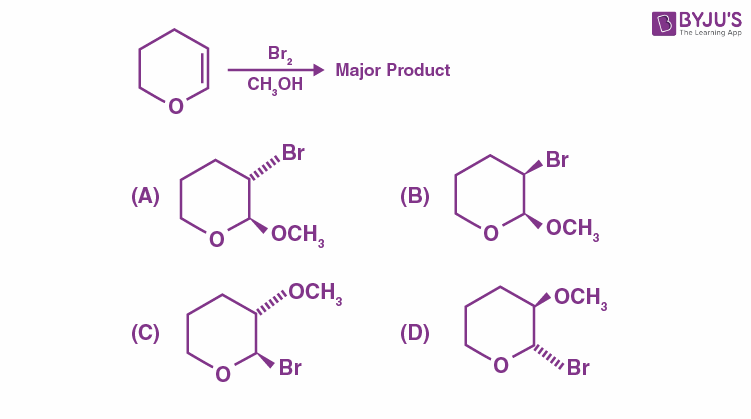Sol.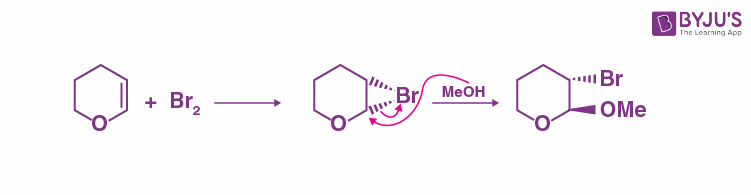16. In the given reaction‘A’ can be

(A) Benzyl bromide

(B) Bromo benzene

(C) Cyclohexyl bromide

(D) Methyl bromide

Sol.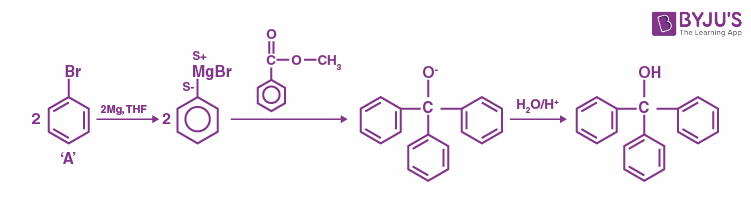Hence ‘A’ is bromobenzene.

17. Which of the following conditions or reaction sequence will NOT give acetophenone as the major product?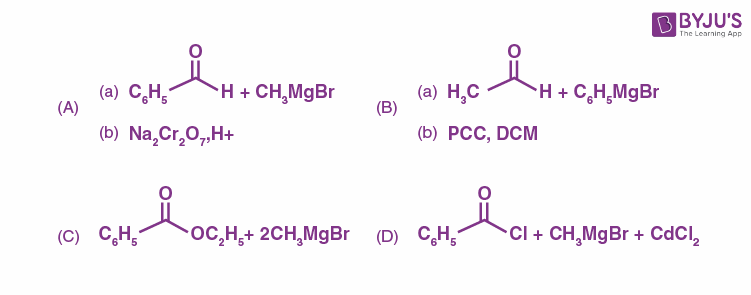Sol. C will not give acetophenone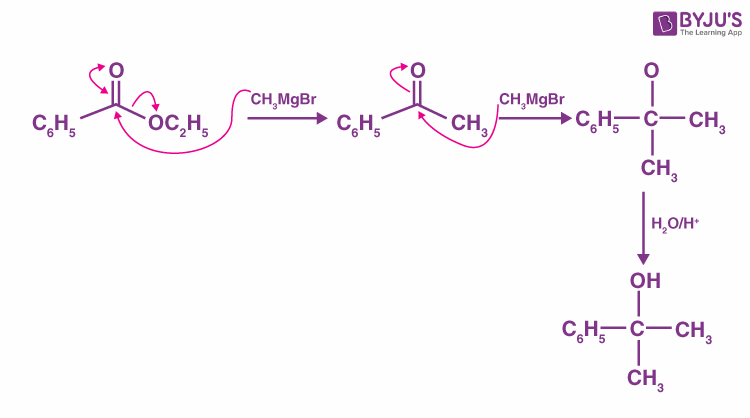18. The major product formed in the following reaction, is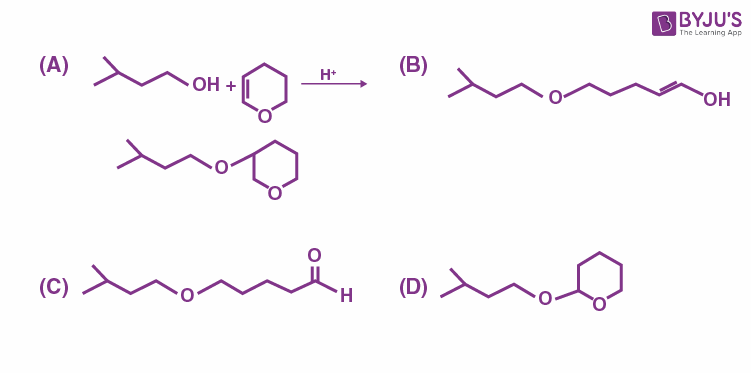Sol.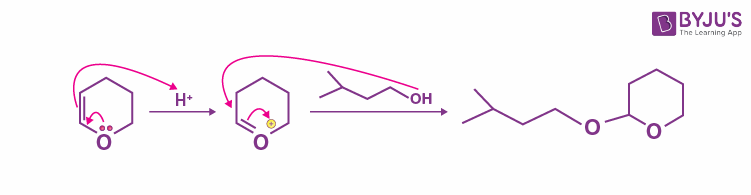19. Which of the following ketone will NOT give enamine on treatment with secondary amines? [where t-Bu is –C(CH3)3]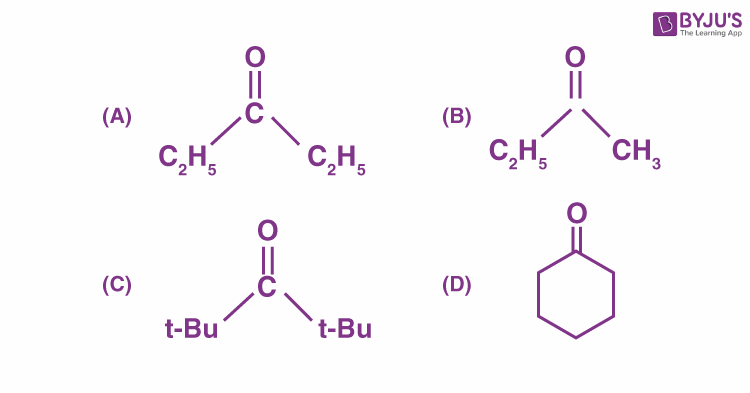Sol. In order to form enamine from the reaction of carbonyl compound with 2º amine, the carbonyl compound must have α-hydrogen.

InNo α-hydrogen is present.

Along with this, due to steric crowding by t-Bu group, it is difficult for 2º amine to attack on this compound.

20. An antiseptic Dettol is a mixture of two compounds ‘A’ and ‘B’ where A has 6π electrons and B has 2π electrons. What is ‘B’?

(A) Bithionol

(B) Terpineol

(C) Chloroxylenol

(D) Chloramphenicol

Sol. Dettol is a mixture of chloroxylenol and terpineol. Chloroxylenol has 6π electrons and terpineol has 2π electrons.

Hence B is terpineol.

#### SECTION – B

Numerical Value Type Questions: This section contains 10 questions. In Section B, attempt any five questions out of 10. The answer to each question is a NUMERICAL VALUE. For each question, enter the correct numerical value (in decimal notation, truncated/rounded-off to the second decimal place; e.g. 06.25, 07.00, –00.33, –00.30, 30.27, –27.30) using the mouse and the on-screen virtual numeric keypad in the place designated to enter the answer.

1. A protein ‘A’ contains 0.30% of glycine (molecular weight 75). The minimum molar mass of the protein ‘A’ is _______ × 103 g mol–1 [nearest integer]

Sol. 0.3% glycine means

100 g protein ‘A’ contains 0.3 g glycine.

Since, molar mass of glycine is 75.

75 g glycine will be present in

$$\begin{array}{l}\frac{100}{0.3}\times75\ g\end{array}$$
protein

Minimum molar mass of protein A is 25 × 103 g/mol

2. A rigid nitrogen tank stored inside a laboratory has a pressure of 30 atm at 06:00 am when the temperature is 27°C. At 03:00 pm, when the temperature is 45°C, the pressure in the tank will be _______ atm. [nearest integer]

Sol. Since

P ∝ T

Hence,

$$\begin{array}{l}\frac{P_1}{T_1}=\frac{P_2}{T_2}\end{array}$$
$$\begin{array}{l}\begin{pmatrix}P_1 \text{is pressure at 6 am} \\P_2 \text{is pressure at 3 pm}\end{pmatrix}\end{array}$$
$$\begin{array}{l}\frac{30}{300}=\frac{P_2}{318}\end{array}$$

P2 ≃32 atm

3. Amongst BeF2, BF3, H2O, NH3, CCl4 and HCl, the number of molecules with non-zero net dipole moment is ______.

Sol.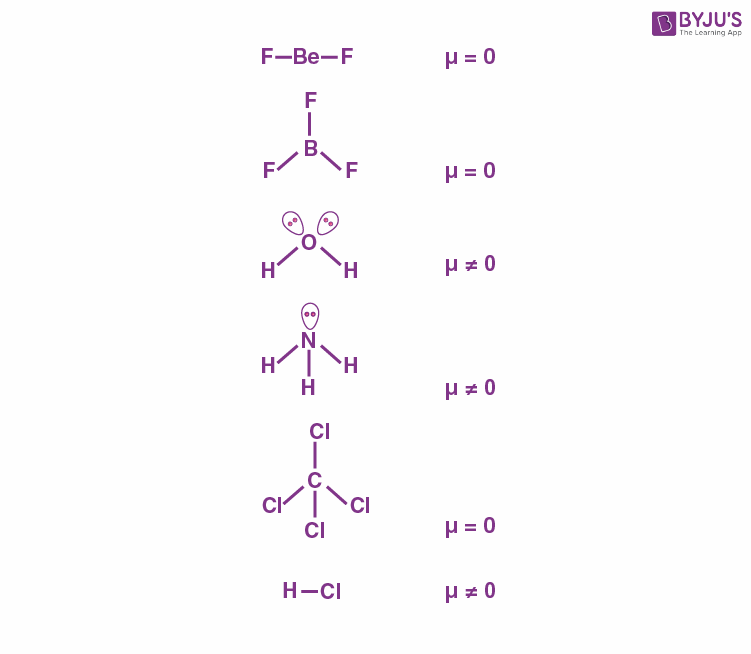4. At 345 K, the half life for the decomposition of a sample of a gaseous compound initially at 55.5 kPa was 340 s. When the pressure was 27.8 kPa, the half life was found to be 170 s. The order of the reaction is ________. [integer answer]

Sol.

$$\begin{array}{l}t_{1/2}\propto \frac{1}{\left [P_0\right]^{n-1}} \end{array}$$
$$\begin{array}{l}\frac{\left ( t_{1/2}\right )_1}{\left(t_{1/2}\right )_2}=\frac{\left [P_0 \right ]_{2}^{n-1}}{\left [ P_0 \right ]_{1}^{n-1}}\end{array}$$
$$\begin{array}{l}\frac{340}{170}=\left ( \frac{27.8}{55.5} \right )^{n – 1}\end{array}$$
$$\begin{array}{l}2=\left ( \frac{1}{2} \right )^{n-1}\end{array}$$

2 = (2)1 – n

1 – n = 1

n = 0

5. A solution of Fe2(SO4)3 is electrolyzed for ‘x’ min with a current of 1.5 A to deposit 0.3482 g of Fe. The value of x is _______. [nearest integer]

Given : 1 F = 96500 C mol–1

Atomic mass of Fe = 56 g mol–1

Sol. Fe3+ + 3e → Fe

Moles of Fe deposited

$$\begin{array}{l}=\frac{0.3482}{56}=6.2\times10^{-3}\end{array}$$

For 1 mole Fe, charge required is 3 F

For 6.2 × 10–3 mole Fe, charge required is

3 × 6.2 × 10–3 F

Since, charge required = 18.6 × 10–3 × 96500 C

= 1794.9 C

And,

1.5 × t = 1794.9

$$\begin{array}{l}t=\frac{1794.9}{1.5\times60}~\textup{min}\end{array}$$

t ≃20 min

6. Consider the following reactions:

$$\begin{array}{l}PCl_3+H_2O\to A+HCl\end{array}$$
$$\begin{array}{l}A+H_2O\to B+HCl\end{array}$$

The number of ionisable protons present in the product B is

Sol.

$$\begin{array}{l}PCl_3+H_2O\to \underset{A}{P(O}H)Cl_2+HCl\end{array}$$
$$\begin{array}{l}P(\underset{A}{OH})Cl_2+H_2O\to P(\underset{B}{OH})_2Cl+HCl\end{array}$$

Hydrogen attached with oxygen are ionisable. Hence number of ionisable protons present in compound B are 2.

7. Amongst FeCl3.3H2O, K3[Fe(CN)6)] and [Co(NH3)6]Cl3, the spin-only magnetic moment value of the inner-orbital complex that absorbs light at shortest wavelength is_______B.M. [nearest integer]

Sol. [Fe(H2O)3Cl3] → Outer-orbital complex

K3[Fe(CN)6] → Inner-orbital complex

[Co(NH3)6]Cl3 → Inner-orbital complex

Since CN is a strong field ligand than NH3. Hence K3[Fe(CN)6] is the inner-orbital complex that absorbs light at shortest wavelength.

Fe(III) → valence shell configuration 3d5

Since CN will do pairing, so unpaired electron = 1

$$\begin{array}{l}\mu=\sqrt{1(1+2)}=\sqrt{3}~\textup{BM}=2~\textup{BM} \end{array}$$

8. The Novolac polymer has mass of 963 g. The number of monomer units present in it are

Sol. Novolac is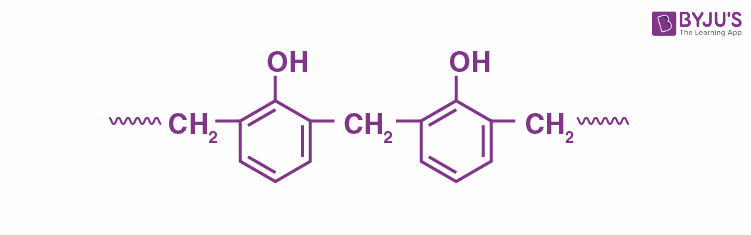Molar mass of monomer is 107 g/mol

$$\begin{array}{l}n=\frac{963}{107}=9\end{array}$$

Number of monomer units present in it are 9.

9. How many of the given compounds will give a positive Biuret test_________? Glycine, Glycylalanine, Tripeptide, Biuret.

Sol. Since dipeptides and free amino acids do not give biuret test. Hence glycine and glycylalanine do not give this test.

10. The neutralization occurs when 10 mL of 0.1M acid ‘A’ is allowed to react with 30 mL of 0.05 M base M(OH)2. The basicity of the acid ‘A’ is_________.

[M is a metal]

Sol. Milieq of acid A = Milieq of base M(OH)2

$$\begin{array}{l}(M\times V\times n-Factor)_A=(M\times V\times n-Factor)_{M(OH)_2}\end{array}$$
[n-Factor of M(OH)2 = 2]

0.1 × 10 × n-Factor = 0.05 × 30 × 2

(n-Factor)A = 3

Hence basicity of acid A is 3.

### Download PDF of JEE Main 2022 June 25 Shift 2 Chemistry Paper & Solutions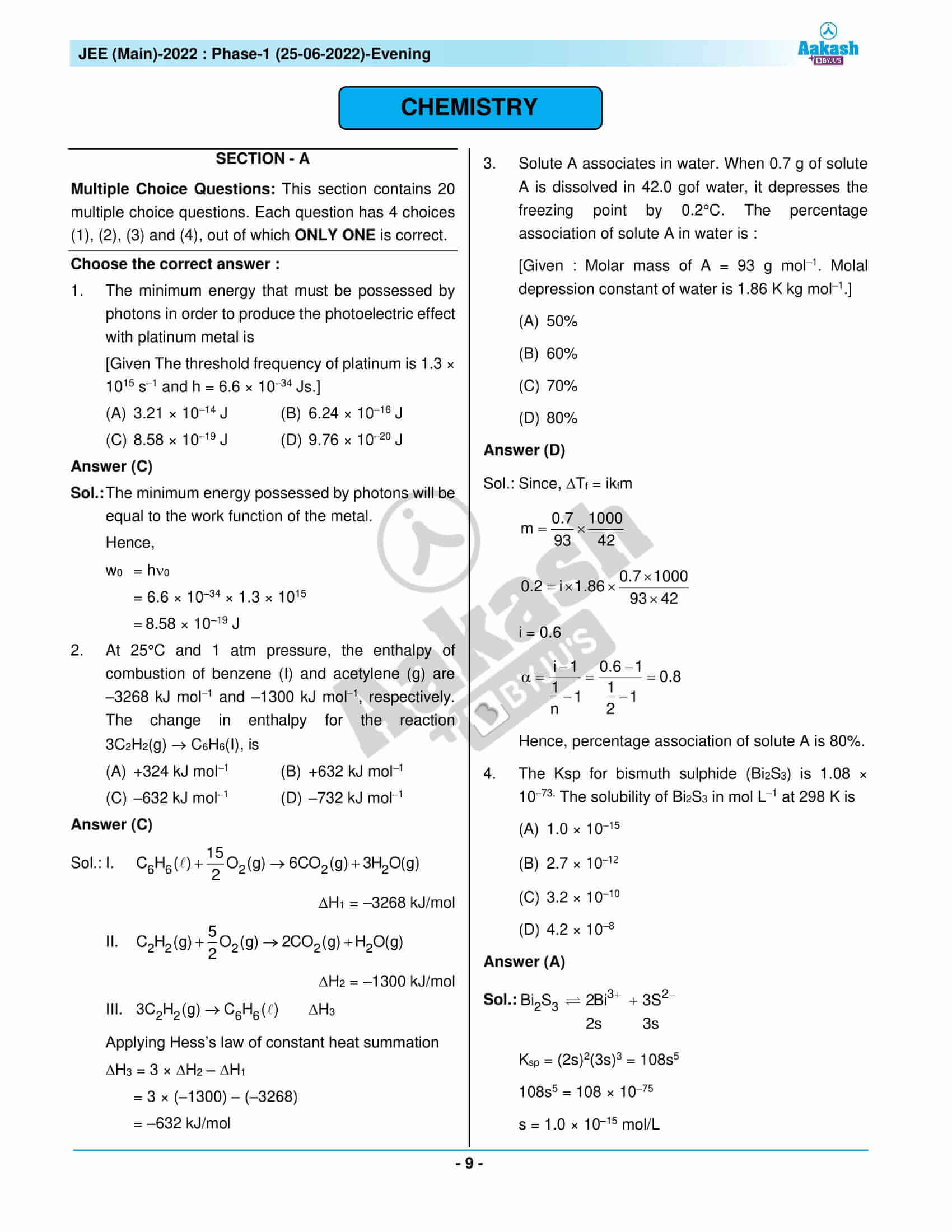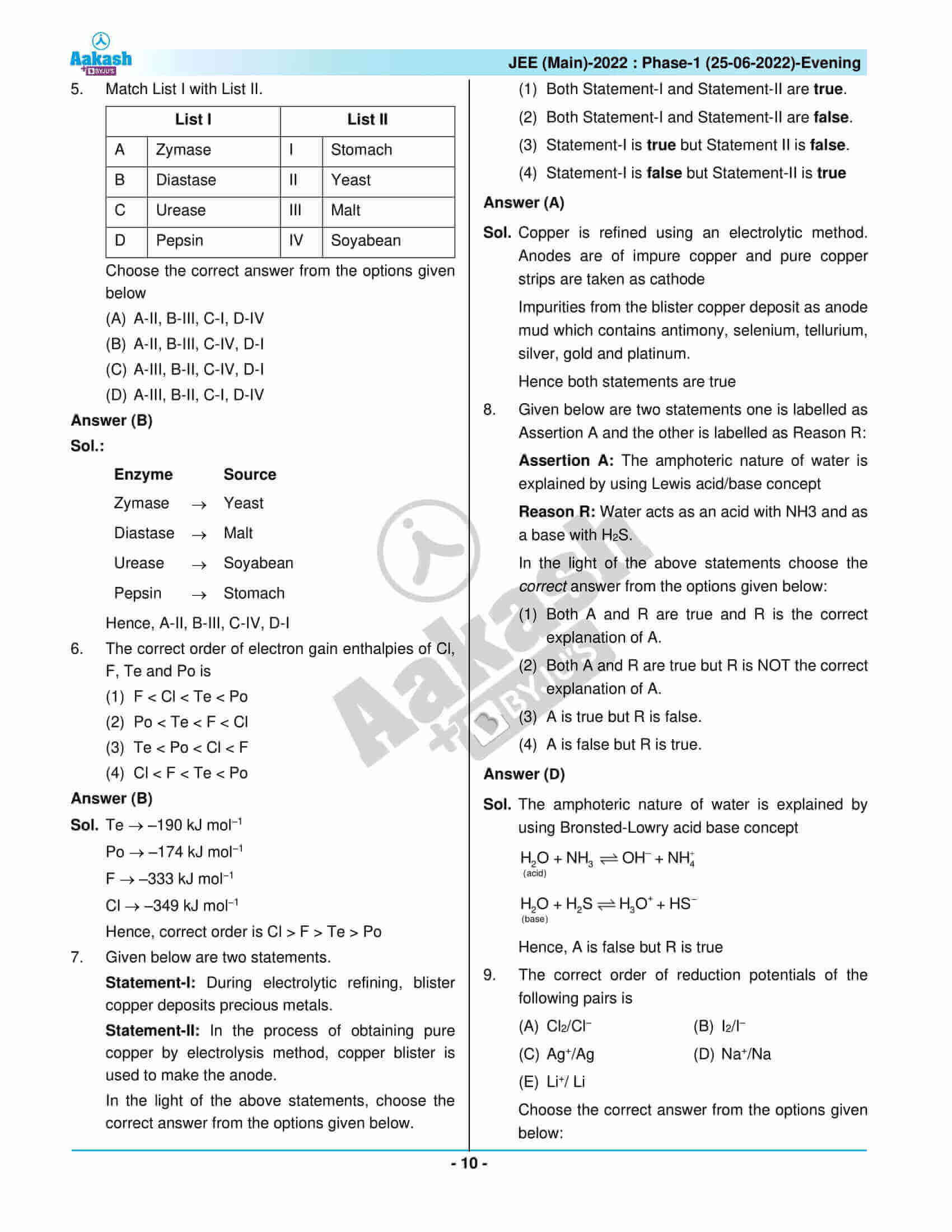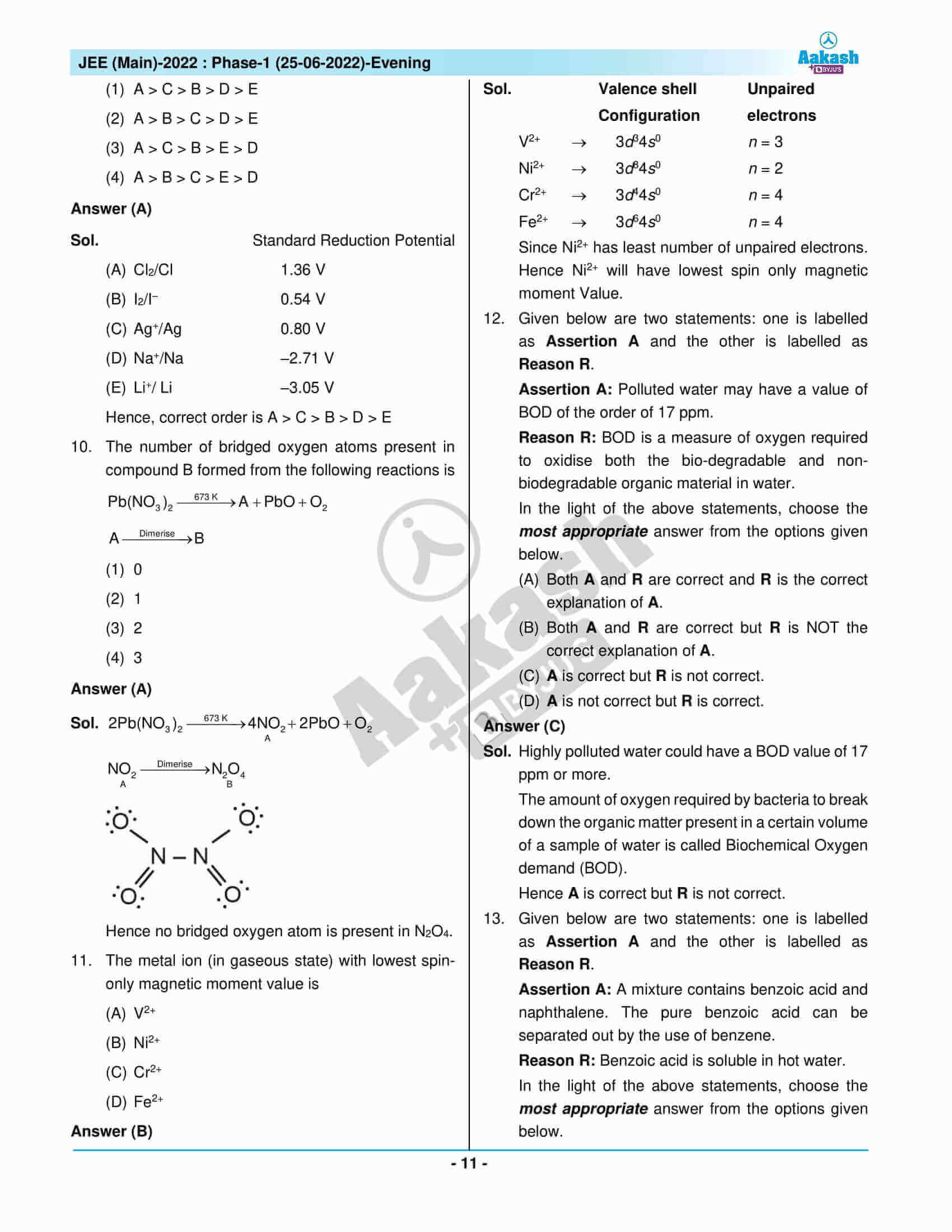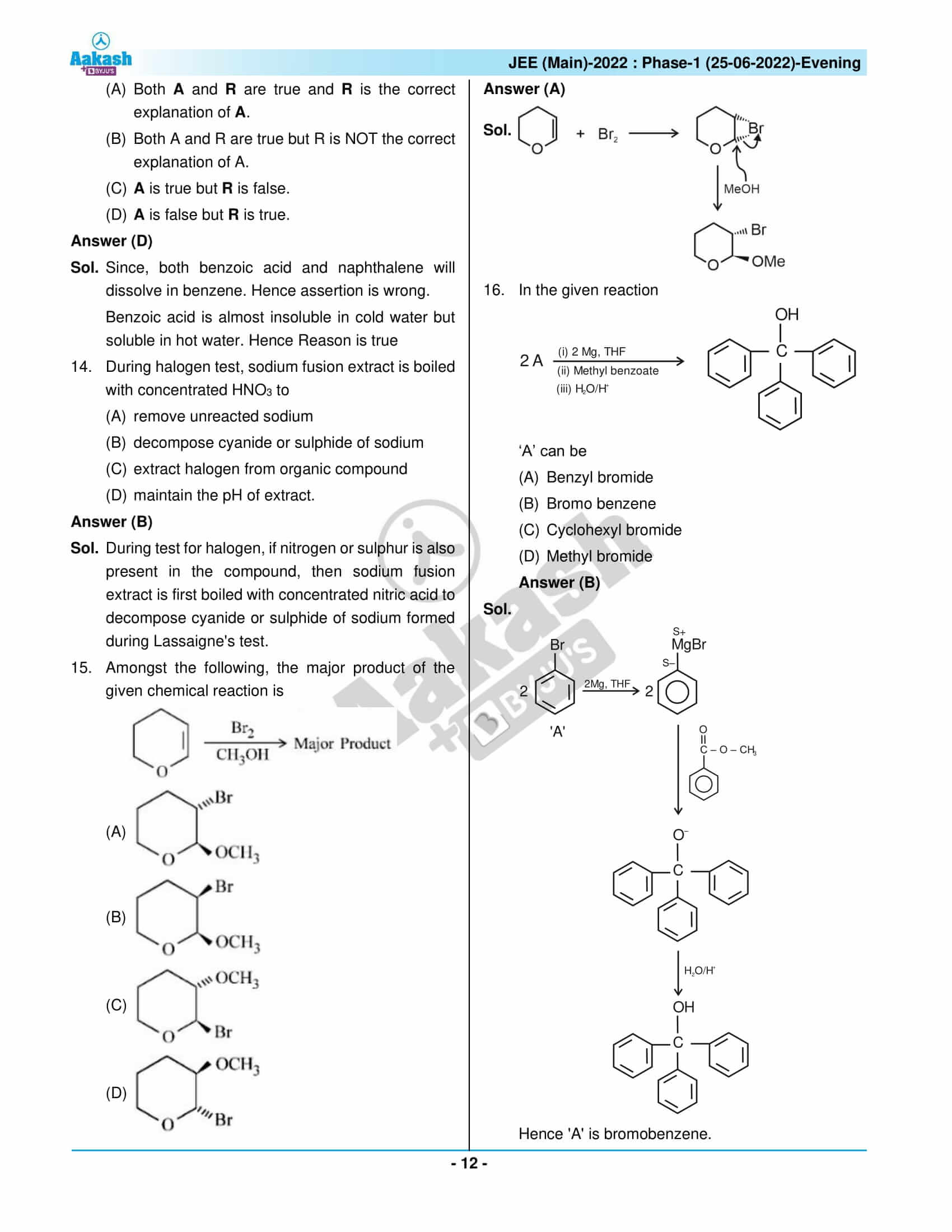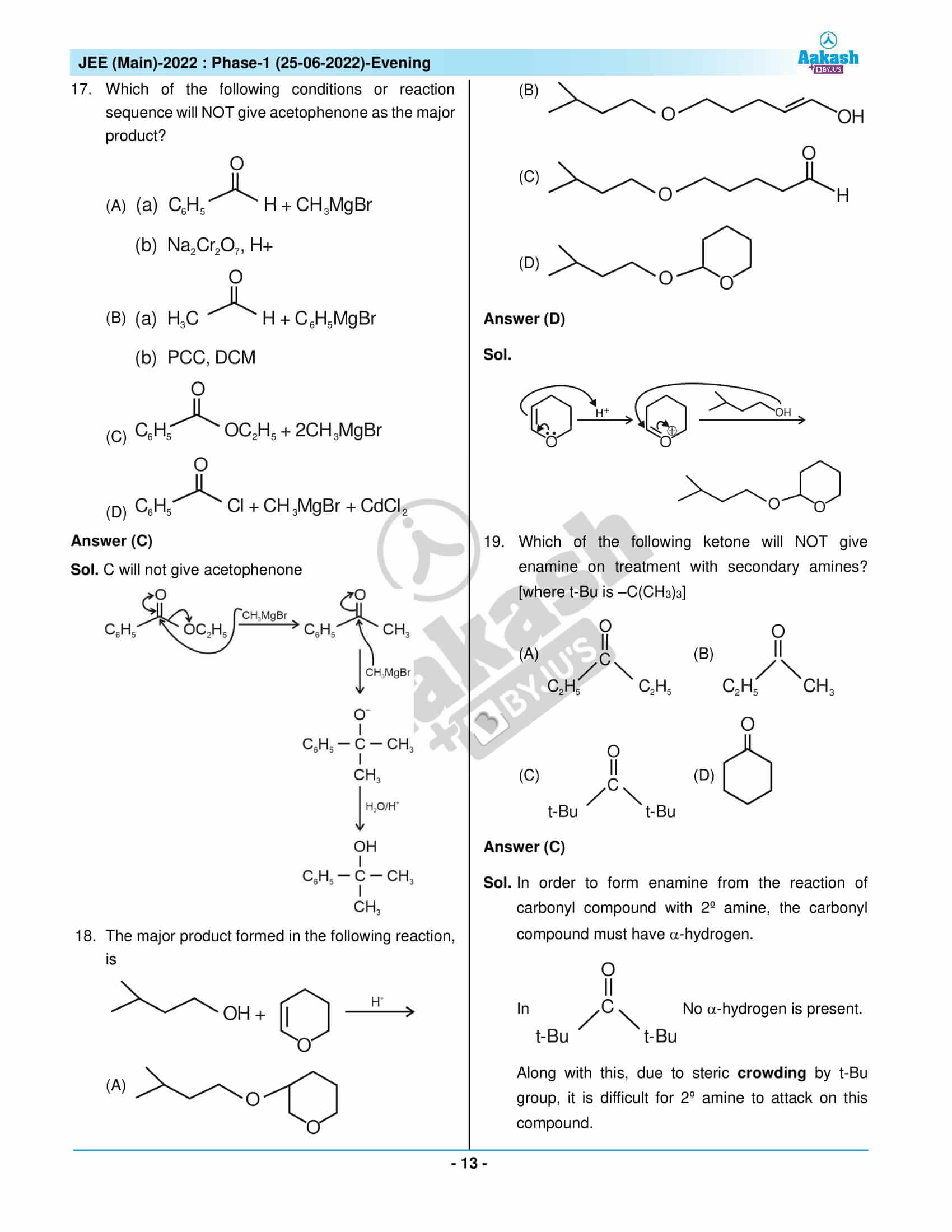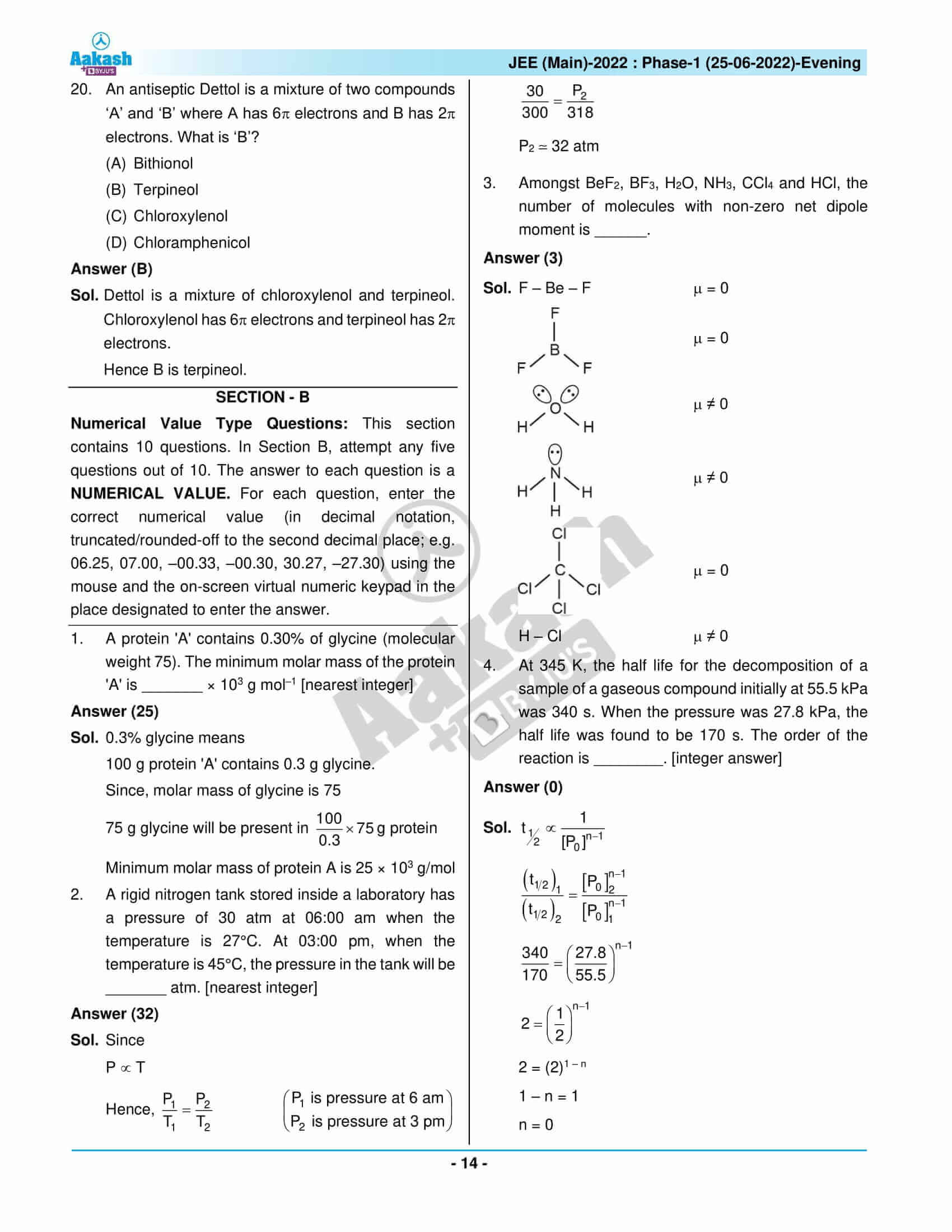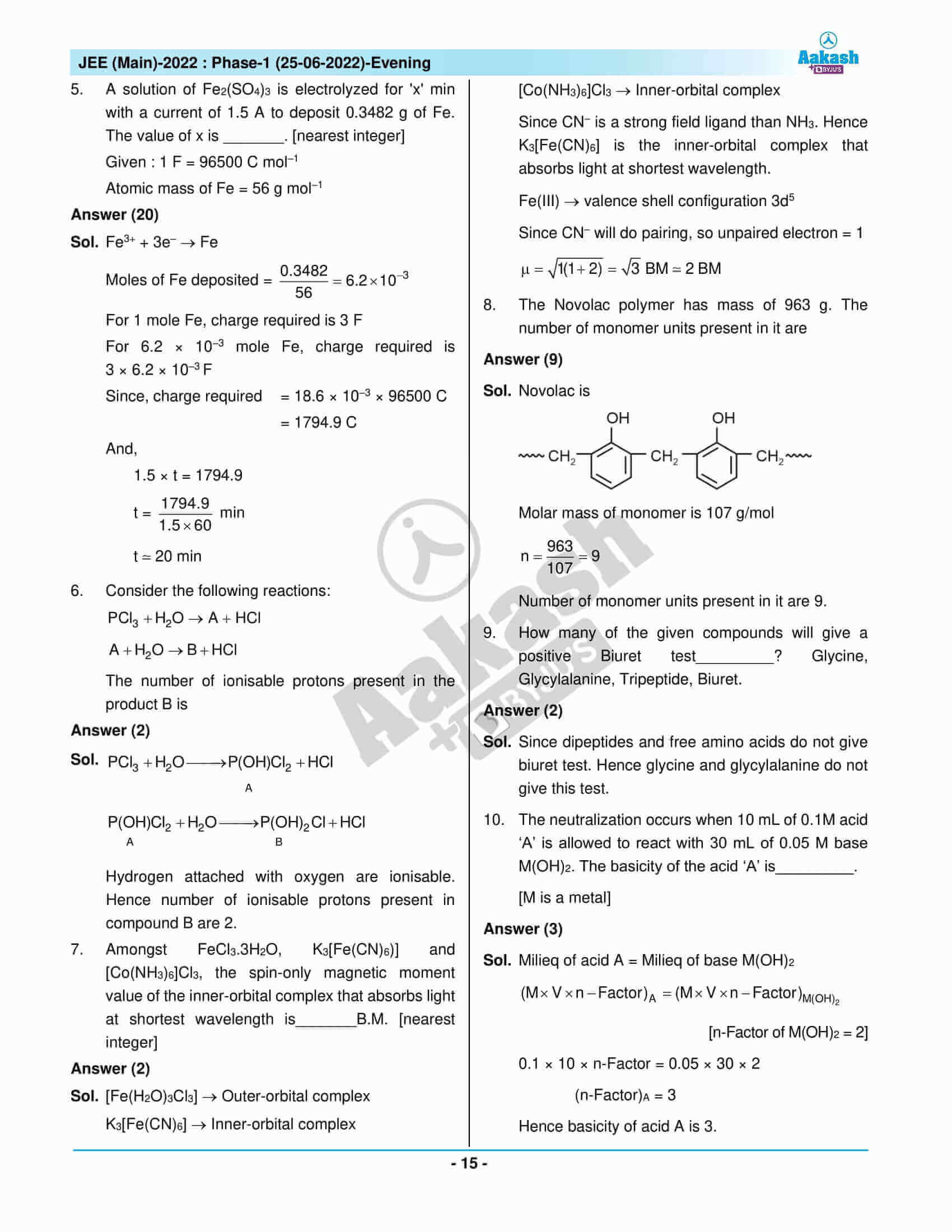## JEE Main 2022 Question Paper with Solutions – June 25 Shift 2

### JEE Main 2022 June 25 Shift 2 Question Paper – Physics Solutions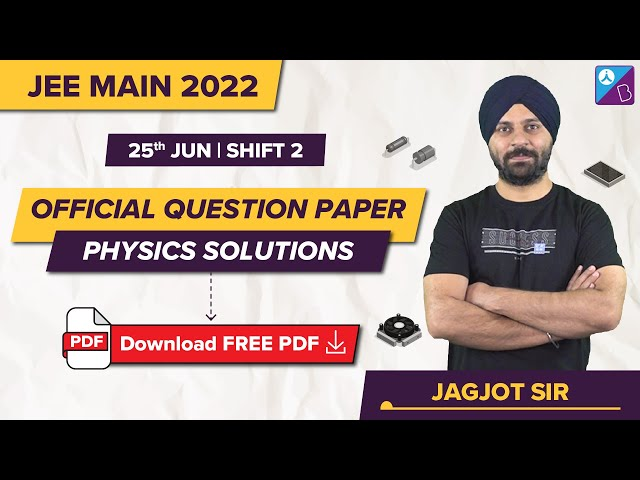### JEE Main 2022 June 25 Shift 2 Question Paper – Chemistry Solutions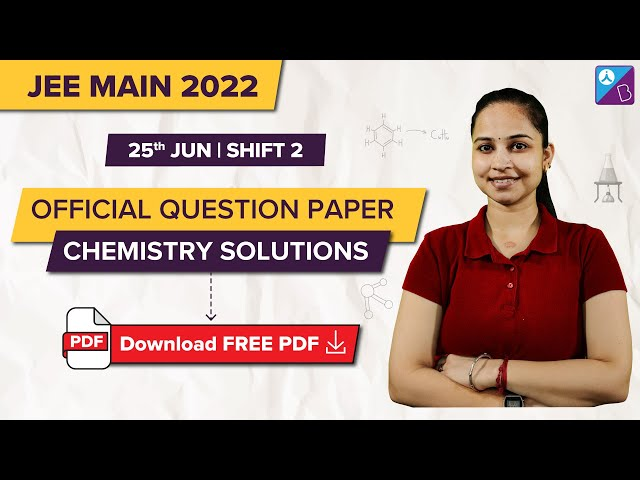### JEE Main 2022 June 25 Shift 2 Question Paper – Maths Solutions## Frequently Asked Questions – FAQs

Q1

### What is the difficulty level of the Chemistry questions in JEE Main 2022 June 25 shift 2 question paper?

Most of the questions from Chemistry were either easy or of moderate difficulty, and quite a few were difficult. The difficulty level of the Chemistry questions is rated 1.73 on a scale of 1 to 3.

Q2

### What was the difficulty level of Chemistry questions compared to Physics and Maths questions in JEE Main 25th June 2022 shift 2 question paper?

Chemistry was the hardest out of the three subjects. Chemistry had 15 medium and 9 easy questions & 2 difficult questions out of the 26 memory-based questions.

Q3

### What are the total marks allotted for Chemistry questions in the June 25 shift 2 JEE Main question paper 2022?

A total of 120 marks is allotted for each subject.

Q4

### What was the topic asked from the chapter “Structure of Atoms” in the Chemistry session of JEE Main 2022 June 25 evening shift question paper?

The topic asked under the chapter structure of atoms is the quantum mechanical model of the atom.

Q5

### How many Chemistry MCQs are asked in JEE Main?

20 Chemistry MCQs are added in JEE Main. A total of 80 marks is allotted for Chemistry MCQs.